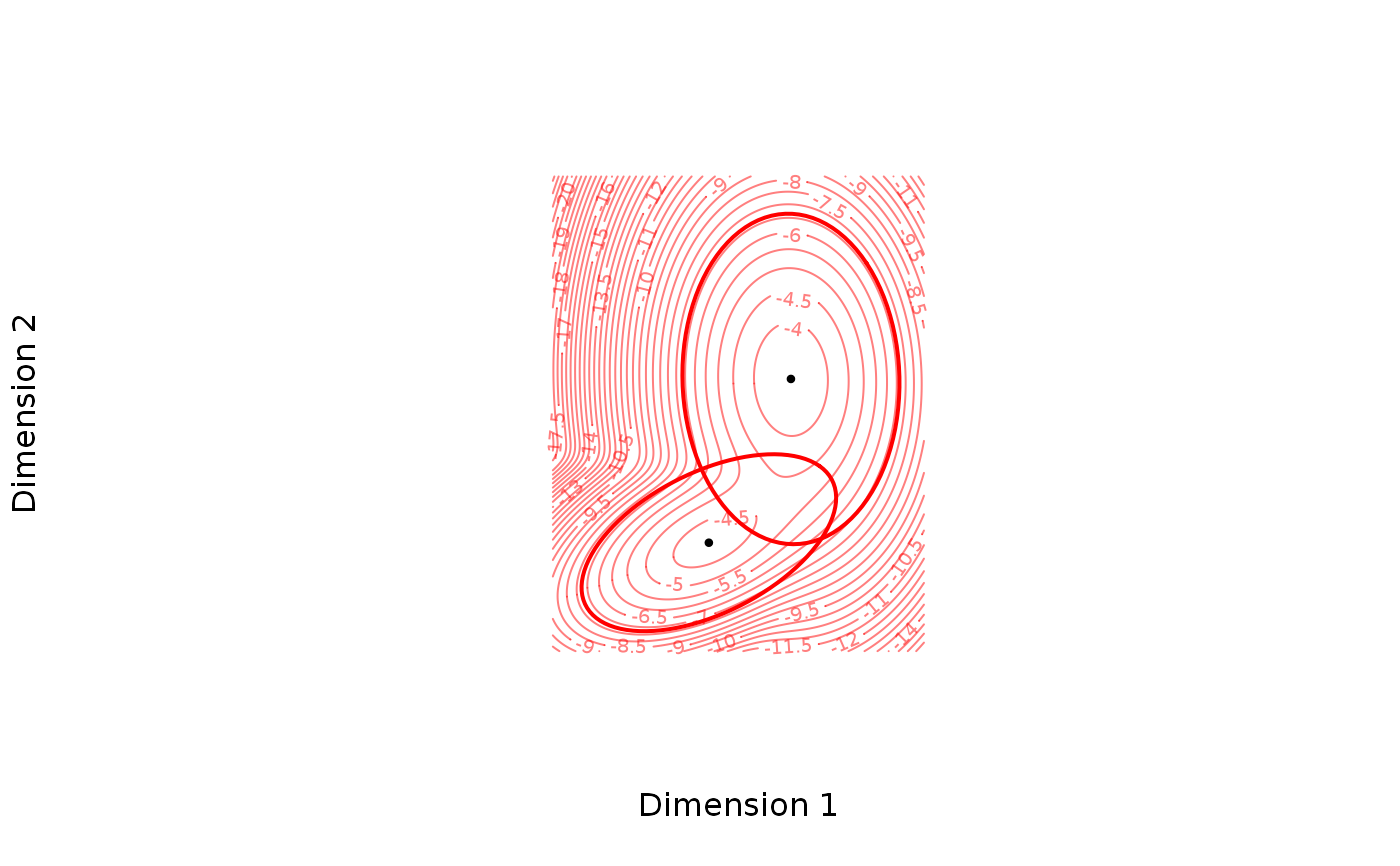Visualizes the chosen dimensions of the theta object graphically by the GMM density and possibly the individual gaussian components.

# S3 method for theta
plot(x, which.dims = c(1L, 2L), n.sd = qnorm(0.99),
add.means = TRUE, ..., add.ellipses = FALSE)

## Arguments

x An object of class theta. An integer vector of length 2 choosing which two dimensions to plot. An integer choosing the number of standard deviations in each dimension to determine the plotting window. logical. If TRUE, dots corresponding to the means are added to the plot. Arguments passed to contour. logical. If TRUE, ellipses outlining a 95% confidence regions for each component are added in the bivariate multivariate distribution defined by theta and which.dims.

## Value

Plots via the contour function. Invisibly returns a list with x, y, z coordinates that is passed to contour.

## Author

Anders Ellern Bilgrau <anders.ellern.bilgrau@gmail.com>

## Examples

set.seed(5)
theta <- rtheta(d = 3, m = 2)
if (FALSE) {
plot(theta)
plot(theta, col = "blue", asp = 1, add.means = FALSE)
plot(theta, col = "blue", asp = 1, add.means = TRUE)
plot(theta, which.dims = c(3L, 2L), asp = 1)
}
plot(theta, asp = 1, n.sd = 3, add.ellipses = TRUE,
nlevels = 40, axes = FALSE,
xlab = "Dimension 1", ylab = "Dimension 2")